Ex 4.3

Chapter 4 Class 9 Linear Equations in Two Variables
Serial order wise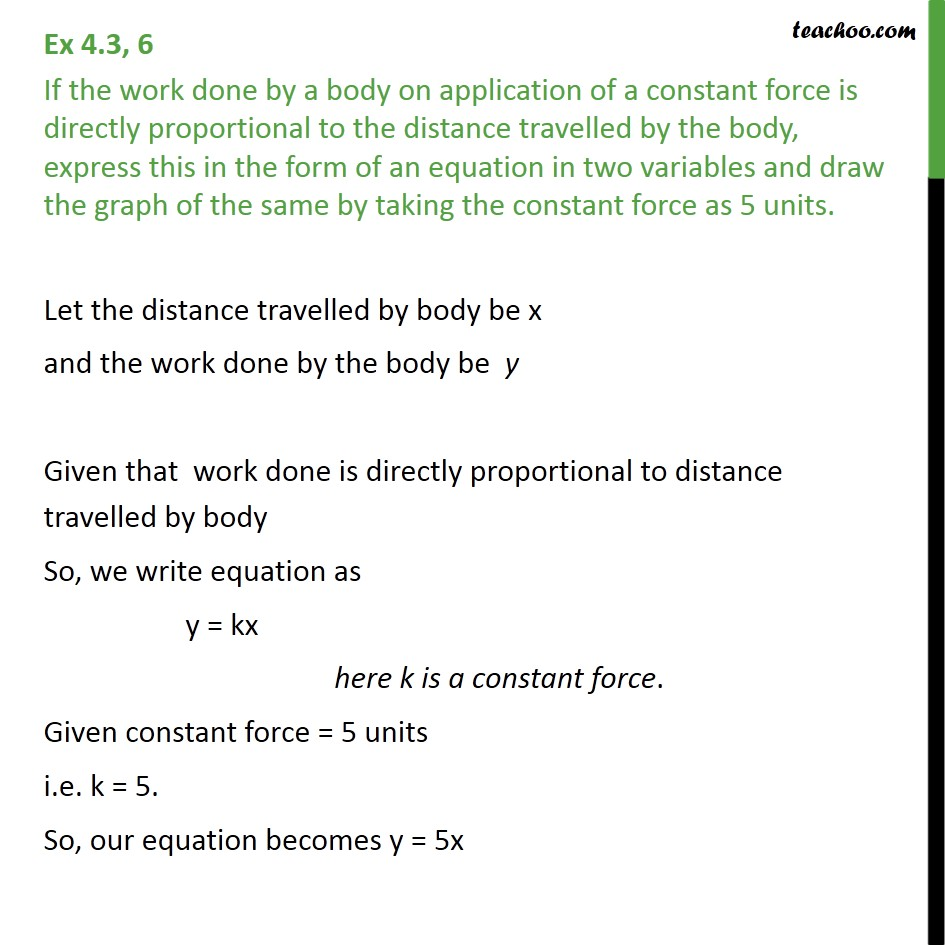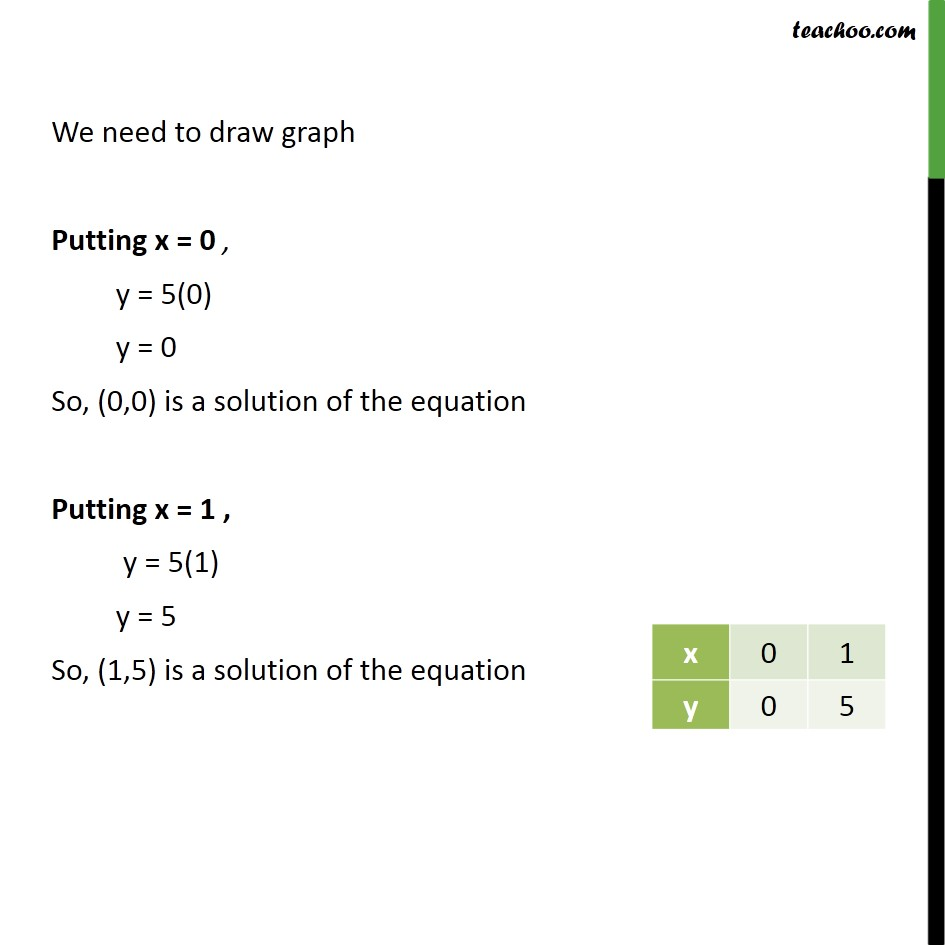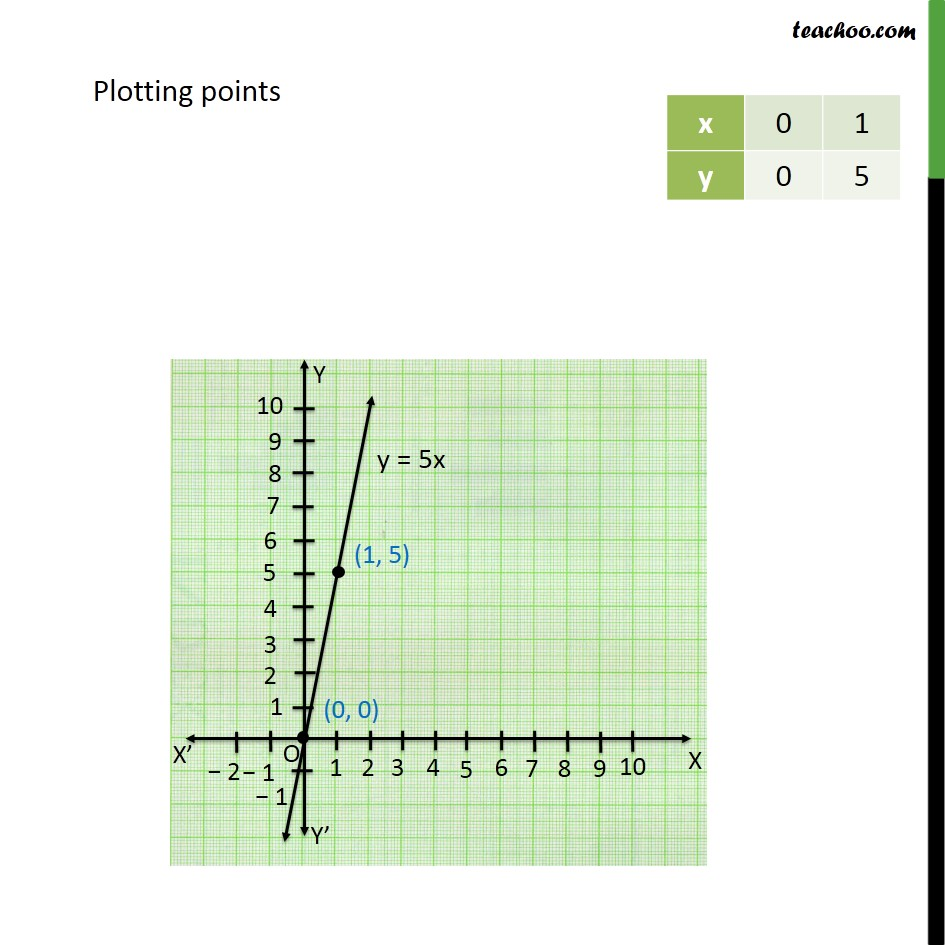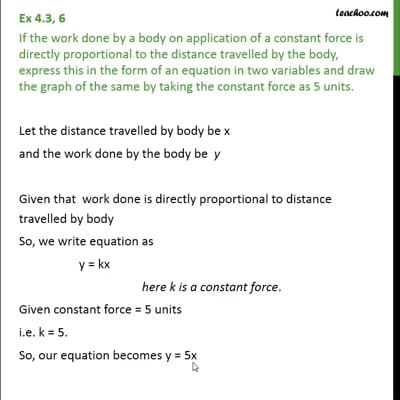This video is only available for Teachoo black users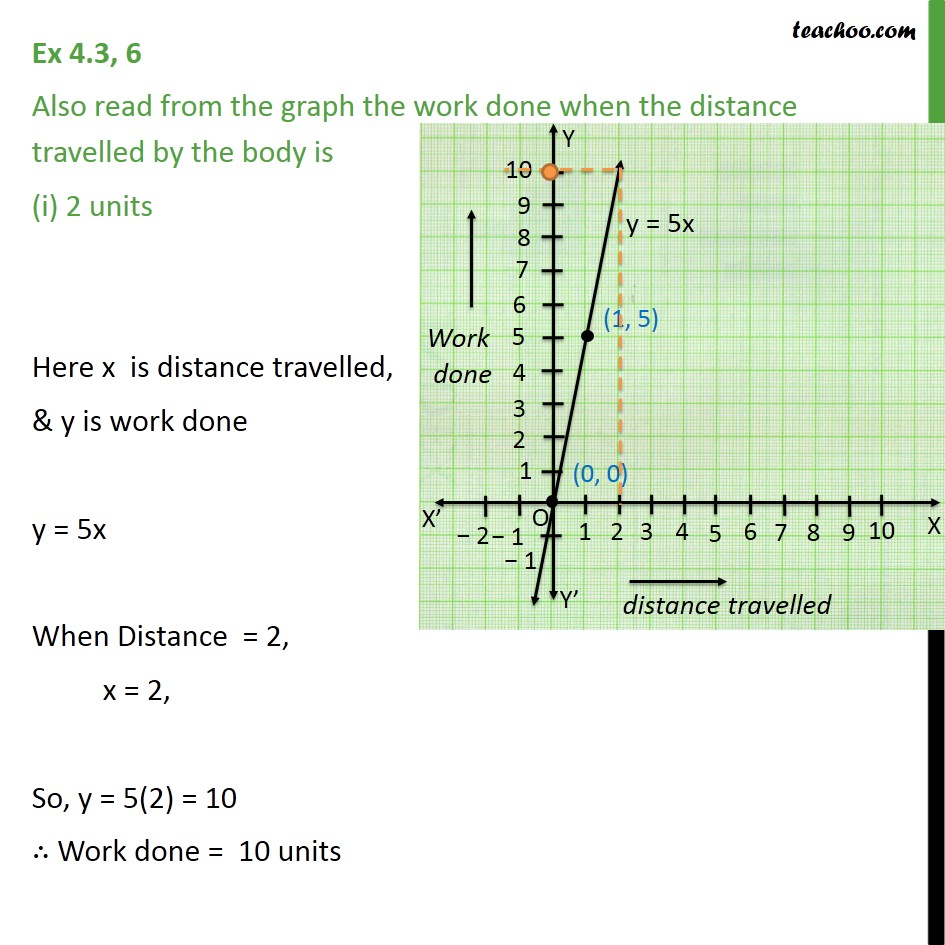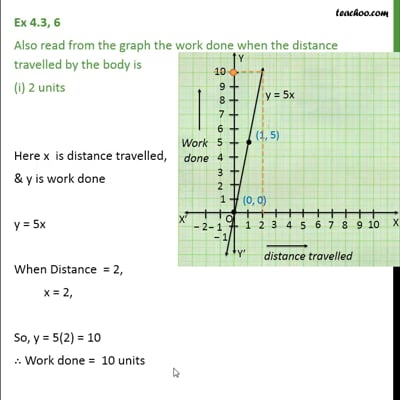This video is only available for Teachoo black users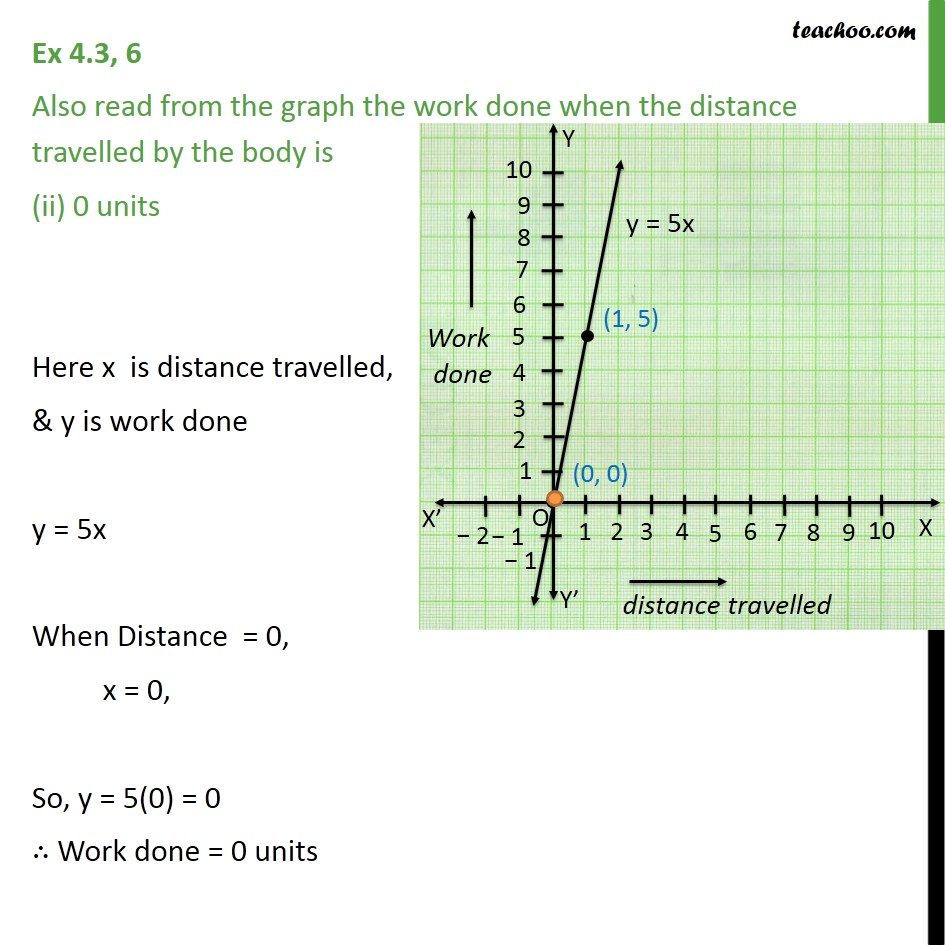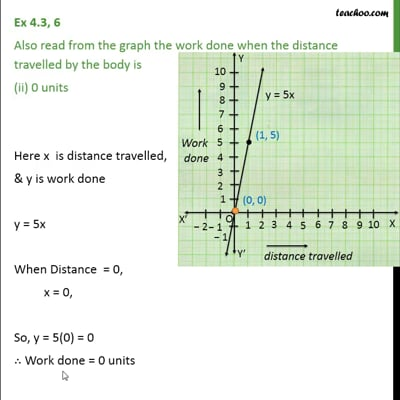This video is only available for Teachoo black users

Solve all your doubts with Teachoo Black (new monthly pack available now!)

### Transcript

Ex 4.3, 6 If the work done by a body on application of a constant force is directly proportional to the distance travelled by the body, express this in the form of an equation in two variables and draw the graph of the same by taking the constant force as 5 units. Let the distance travelled by body be x and the work done by the body be y Given that work done is directly proportional to distance travelled by body So, we write equation as y = kx here k is a constant force. Given constant force = 5 units i.e. k = 5. So, our equation becomes y = 5x We need to draw graph Putting x = 0 , y = 5(0) y = 0 So, (0,0) is a solution of the equation Putting x = 1 , y = 5(1) y = 5 So, (1,5) is a solution of the equation Plotting points Ex 4.3, 6 Also read from the graph the work done when the distance travelled by the body is (i) 2 units Here x is distance travelled, & y is work done y = 5x When Distance = 2, x = 2, So, y = 5(2) = 10 Work done = 10 units Ex 4.3, 6 Also read from the graph the work done when the distance travelled by the body is (ii) 0 units Here x is distance travelled, & y is work done y = 5x When Distance = 0, x = 0, So, y = 5(0) = 0 Work done = 0 units